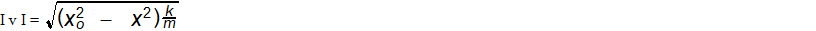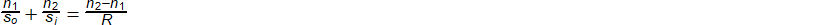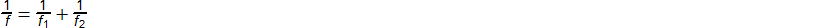### AP Physics 1 Week 4 (July 2 - July 8, 2017)

Part 1:  Algebra (Rearrange)

1.  solve for x2.  solve for m3. solve for n14.  solve for n25.  solve for f2Part 2:  Algebra (Inverse and Direct Relationships)

6.  A bomber-pilot coming out of a dive at a velocity of 600 feet per second experiences a centripetal force of 1800 pounds.  If centripetal force is proportional to the square of velocity, find the force on this pilot flying the same path at 1000 feet per second.  (DBW, p224, #1)

Hint:  Fc is proportional to v2, then Fc/v2 = Fc/v2

7.  The force exerted by the wind on a sail of a fixed area varies directly (proportional to) as the square of the wind velocity.  If the force exerted on a given sail is 180 pounds when the wind is blowing 15 miles per hour, what is the velocity of the wind when the force on the sail is 500 pounds?  (DBW, p224, #2)

8.  The energy of a 2 pound satellite orbiting 17,500 miles per hour is equivalent to 27,000 British thermal units of heat.  If the amount of heat varies directly (proportional to) as the square of the velocity, find the heat equivalent of the energy of this satellite orbiting at 25,000 miles per hour.  (DBW, p224, #3)

9.  The power expended by heat in an electric circuit of fixed resistance is directly proportional to the square of the current.  If a circuit expends 180 Watts when a current of 6 amperes is flowing, what is the heat expended when the current is 10 amperes?

(DBW, p224, #4)

10.  The strength of a horizontal beam of rectangular cross section and fixed width and length varies as the square of the thickness.  If a thickness of 6 inches is required to support a weight of 5400 pounds, how thick should a beam be to support a weight of 8000 pounds?  (DBW, p224, #5)

Part 3: Geometry/Trigonometry

(For Physics, make sure your calculator is set in degrees)

In Physics, a vector is a quantity (number) that has both magnitude (units) and direction (usually shown as an angle in degrees).  In the simplest case, the magnitude is the distance from the point (0,0) to the coordinate, which can easily be found using the Pythagorean Theorem.  The direction or angle of the vector is almost always given in respect to the x axis or (east-west) direction.  For these problems, (0,0) will always be the first point.  If the other point were (5,7), the distance from (0,0) to (5,7) or magnitude of the vector would be found using the Pythagorean theorem.  So, the magnitude would be the square root of 74, or 8.602.  We always like to leave answers in decimal form in Physics.  The direction or angle is found by finding the inverse tangent of the y coordinate divided by the x coordinate.  So, the inverse tangent of 5/7 would be 35.53 degrees in reference to the x axis.

With (0,0) as the initial coordinate, find the magnitude and direction of a vector going to each of the points listed below.  The unit for all numbers in given in meters.

11.  (8,4)

12.  (14,24)

13.  (-10, 8)

14.  (7,-15)

15. (-13,-18)

Part 4:  Word Problems

16. An airplane pilot has 1 hour and 10 minutes to go 100 miles and return.  When

going forward she has a tail wind of 20 mph, and returning she has a head wind

of 20 mph.  She estimates she will lose 2.5 minutes of flying time in takeoffs and

landings.  At what air speed must she fly?  (WKS, p275 #8)

17. An airplane makes a trip of 600 miles and returns.  Its speed going was 3/4 the rate

returning.  If it took 1 hour longer to go than to return, what was the speed each

way? (WKS, p364, #27)

18. Bryan and David start at the same time from the same place.  Bryan walks north

and David walks east.  At the end of 3 hours, Bryan has walked 3 miles more

than David and they are 15 miles apart.  What is the speed of both men?

(WKS, p365, #37)

19. A newer blue-line train running 12 mph faster than an older red-line train required

2.5 hours less time to travel 600 miles.  Find the speed of each train.

(WKS, p366, #21)

20. An airplane makes a trip of 400 miles and returns.  Its speed going was 4/5 the

speed returning.  If it took 1 hour longer to go than return, what was the rate

each way? (WKS, p367, #9)